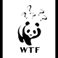Discussion about math, puzzles, games and fun.   Useful symbols: ÷ × ½ √ ∞ ≠ ≤ ≥ ≈ ⇒ ± ∈ Δ θ ∴ ∑ ∫  π  -¹ ² ³ °

You are not logged in.

## #1 2008-05-12 02:26:52

SvenBee
MemberRegistered: 2008-03-08
Posts: 106

### Maxwell's Equations

is the electric field.
is the magnetic field strength.
is the electric displacement field.
is the magnetic flux density.
is the free electric charge density.
is the free current density.
is the differential vector element for surface area A with infinitesimally small magnitude and direction normal to surface S.
is the differential volume V enclosed by surface S.
is the differential vector element of path length tangent to contour C enclosing surface c.
is the instantaneous velocity.
is the divergence operator.
is the curl operator.

[align=center]Differential Form[/align]

Gauss' Law:

Gauss' Law for Magnetism:

Ampere's Law:

[align=center]Integral form[/align]

Gauss' Law:

Gauss' Law for Magnetism: